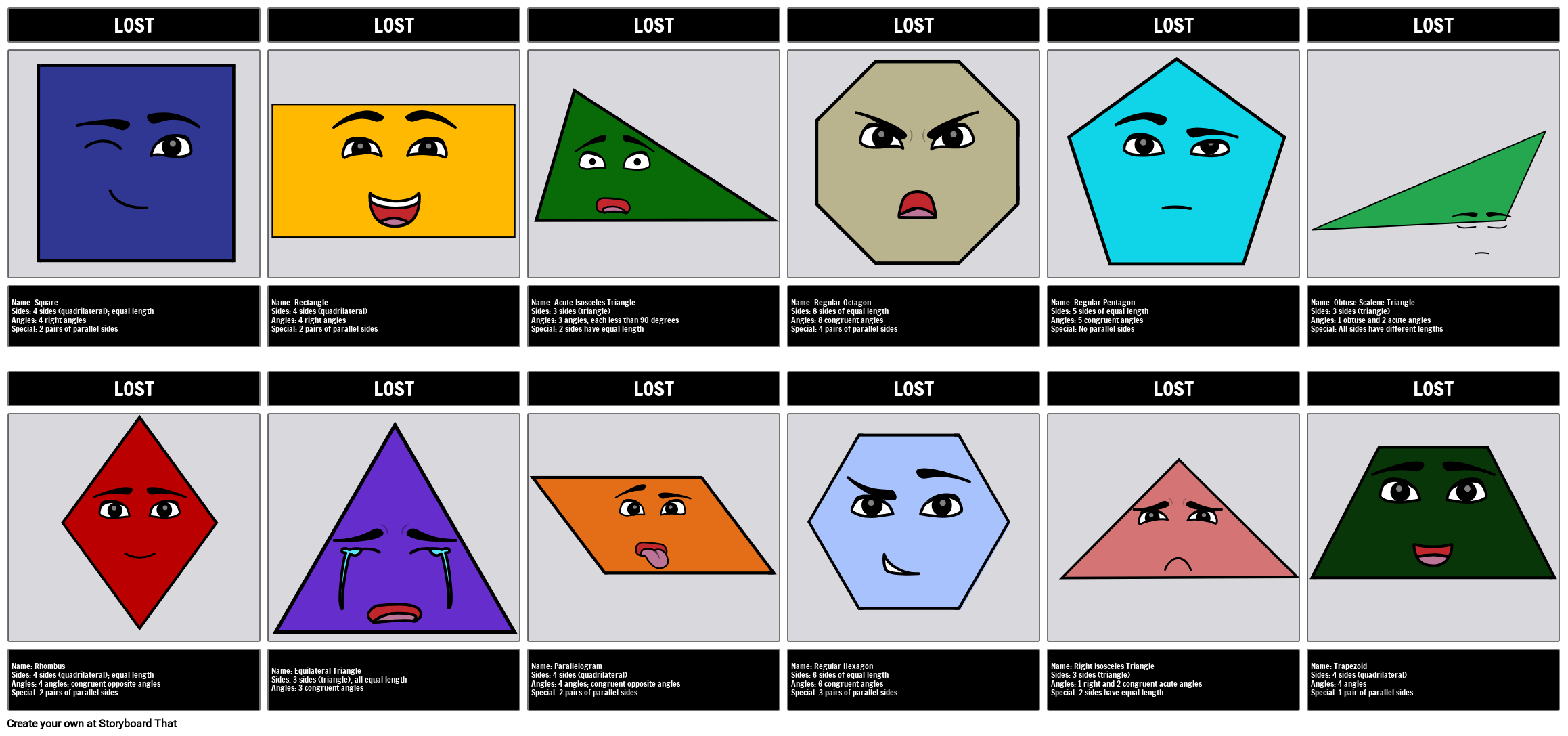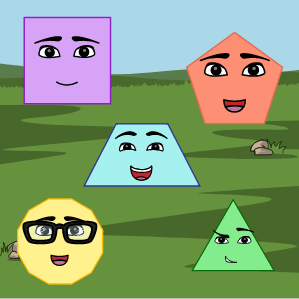# Intro to Geometry - Lost PolygonsYou can find this storyboard in the following articles and resources:### Introduction to Geometry

Lesson Plans by Anna Warfield

In the early grades, students start recognizing, naming, and drawing shapes. As students become more advanced, geometry becomes more complicated. Part of this includes understanding the properties and characteristics of shapes and their relation to one another. The following activities aim to help students master these concepts and prepare them for future geometry lessons.

### Introduction to Geometry

#### Storyboard Description

Introduction to Geometry| Identify common and regular polygons | CCSS.MATH.CONTENT.4.G.A.1Draw points, lines, line segments, rays, angles (right, acute, obtuse), and perpendicular and parallel lines. Identify these in two-dimensional figures. | Introduction to Geometry Lesson Plans | Regular Polygon

#### Storyboard Text

• LOST
• LOST
• LOST
• LOST
• LOST
• LOST
• Name: Square Sides: 4 sides (quadrilateral); equal length Angles: 4 right angles Special: 2 pairs of parallel sides
• LOST
• Name: Rectangle Sides: 4 sides (quadrilateral) Angles: 4 right angles Special: 2 pairs of parallel sides
• LOST
• Name: Acute Isosceles Triangle Sides: 3 sides (triangle) Angles: 3 angles, each less than 90 degrees Special: 2 sides have equal length
• LOST
• Name: Regular Octagon Sides: 8 sides of equal length Angles: 8 congruent angles Special: 4 pairs of parallel sides
• LOST
• Name: Regular Pentagon Sides: 5 sides of equal length Angles: 5 congruent angles Special: No parallel sides
• LOST
• Name: Obtuse Scalene Triangle Sides: 3 sides (triangle) Angles: 1 obtuse and 2 acute angles Special: All sides have different lengths
• LOST
• Name: Rhombus Sides: 4 sides (quadrilateral); equal length Angles: 4 angles; congruent opposite angles Special: 2 pairs of parallel sides
• Name: Equilateral Triangle Sides: 3 sides (triangle); all equal length Angles: 3 congruent angles
• Name: Parallelogram Sides: 4 sides (quadrilateral) Angles: 4 angles; congruent opposite angles Special: 2 pairs of parallel sides
• Name: Regular Hexagon Sides: 6 sides of equal length Angles: 6 congruent angles Special: 3 pairs of parallel sides
• Name: Right Isosceles Triangle Sides: 3 sides (triangle) Angles: 1 right and 2 congruent acute angles Special: 2 sides have equal length
• Name: Trapezoid Sides: 4 sides (quadrilateral) Angles: 4 angles Special: 1 pair of parallel sides
##### Over 20 Million Storyboards Created